Check Full Chapter Explained - Continuity and Differentiability - Continuity and Differentiability Class 12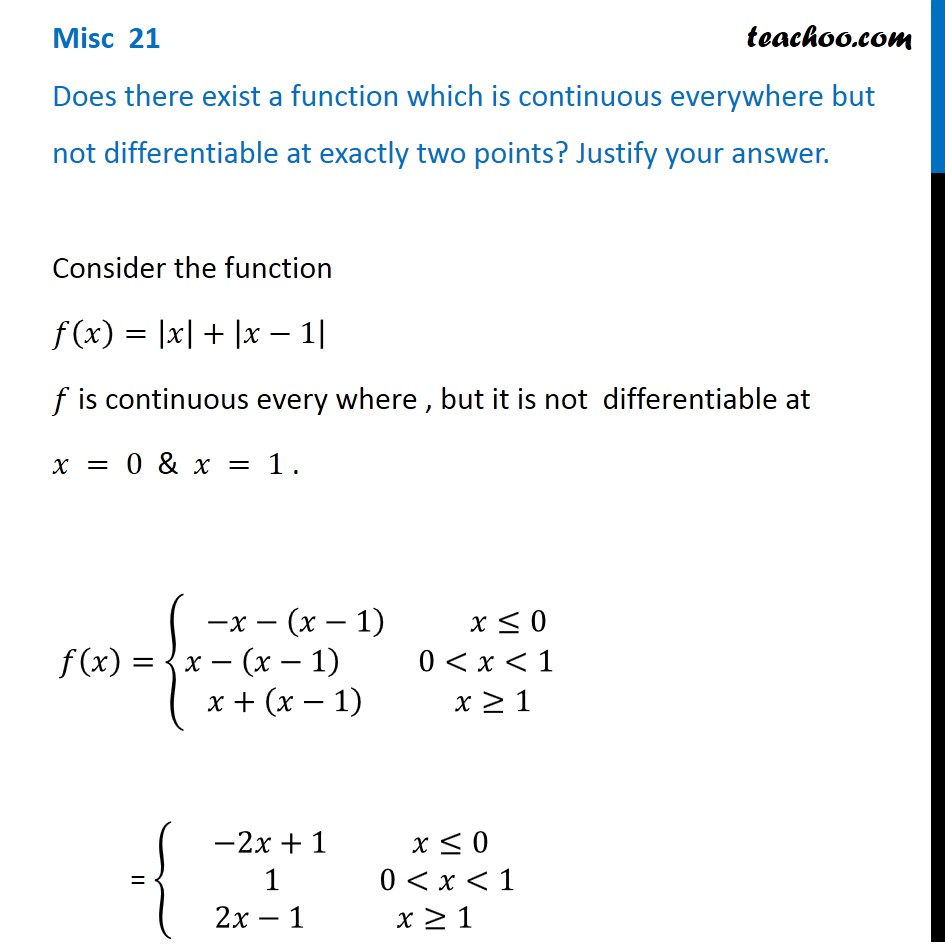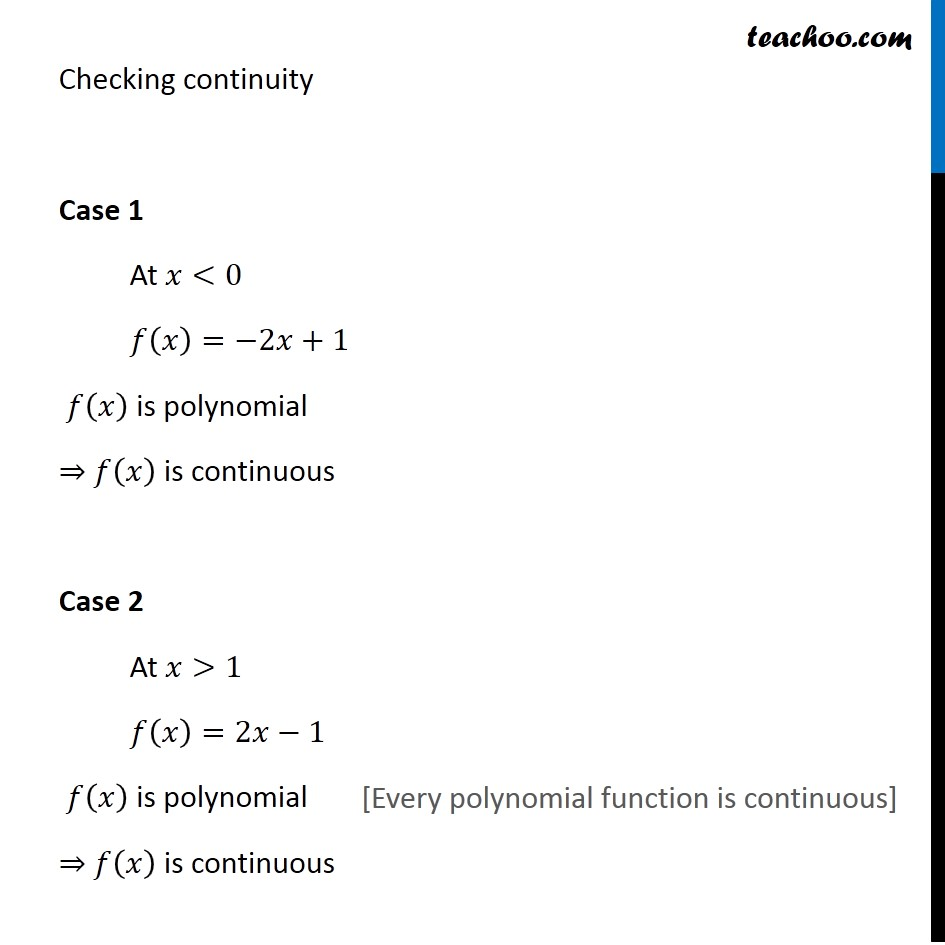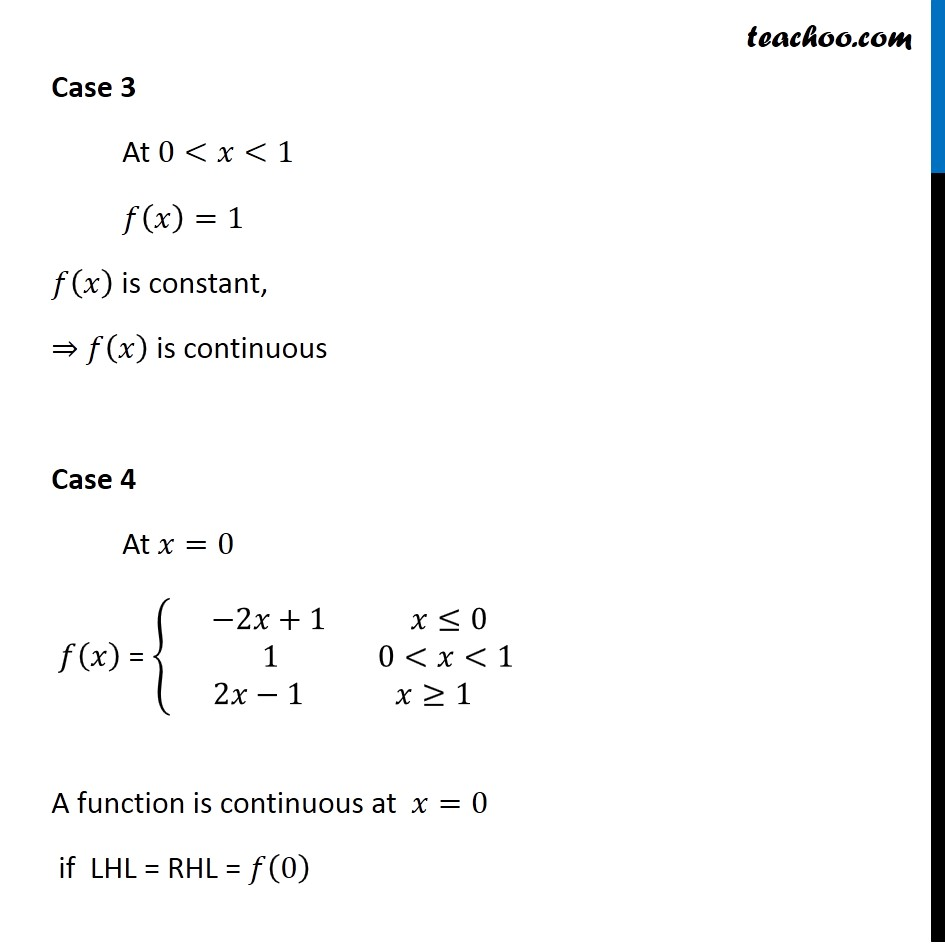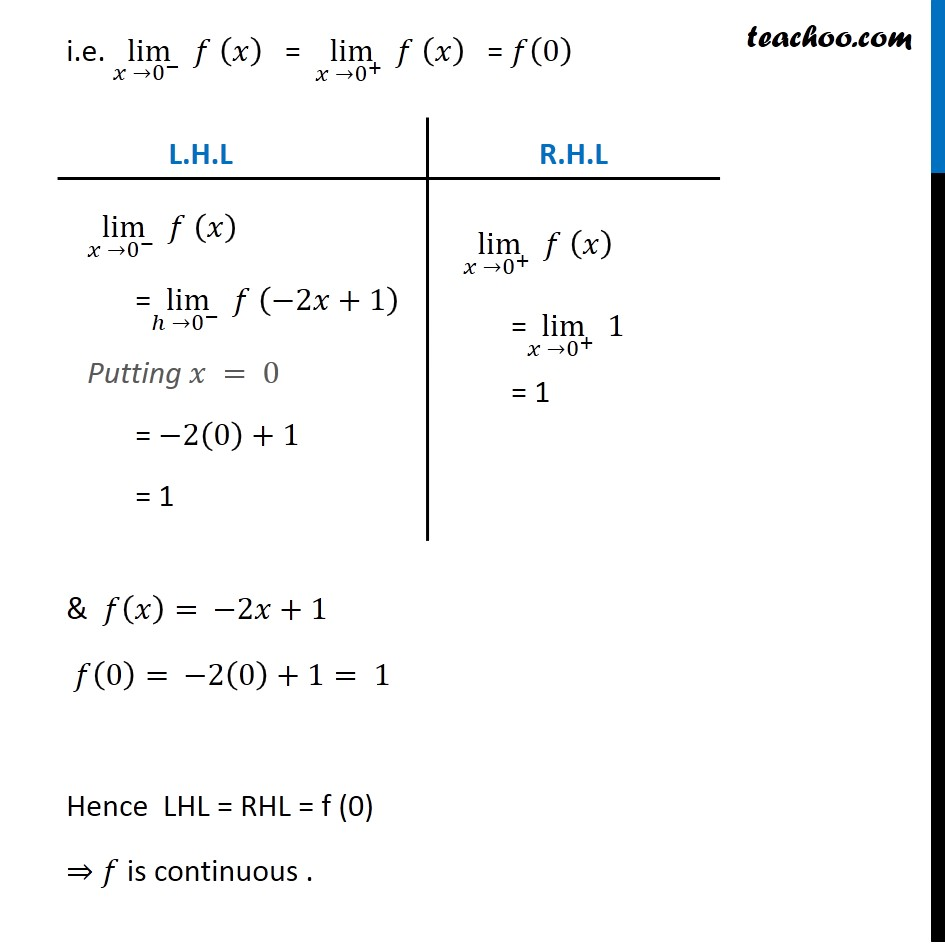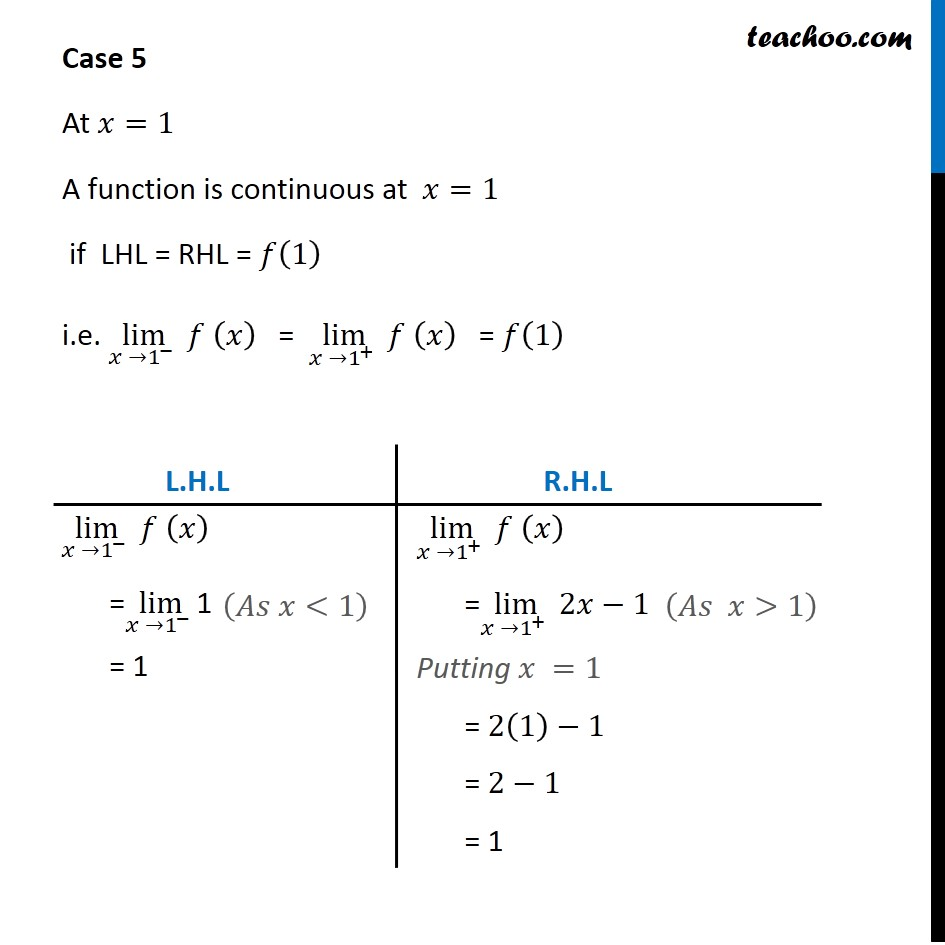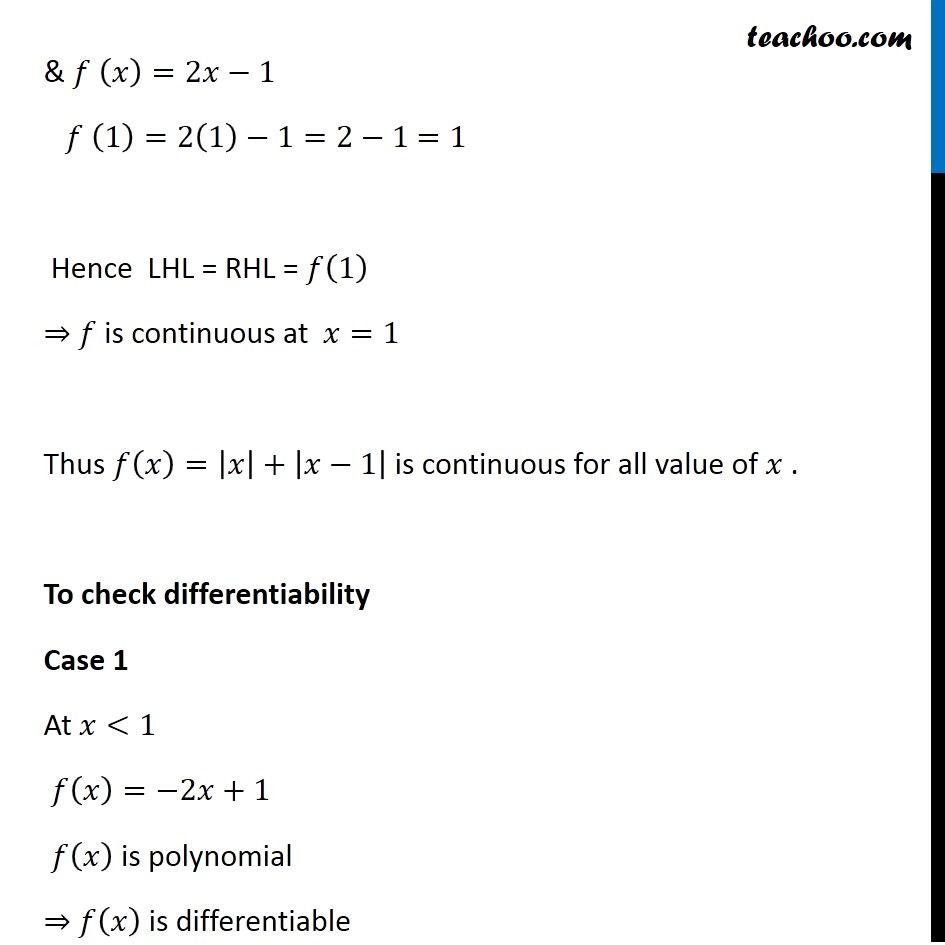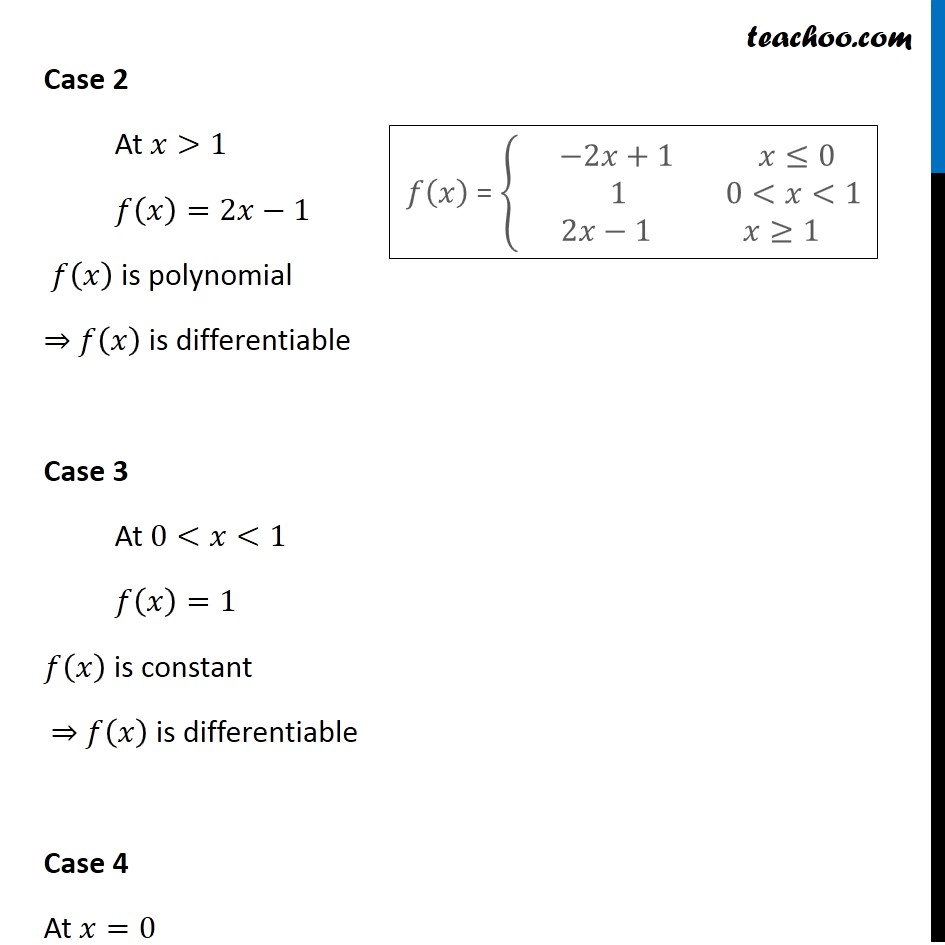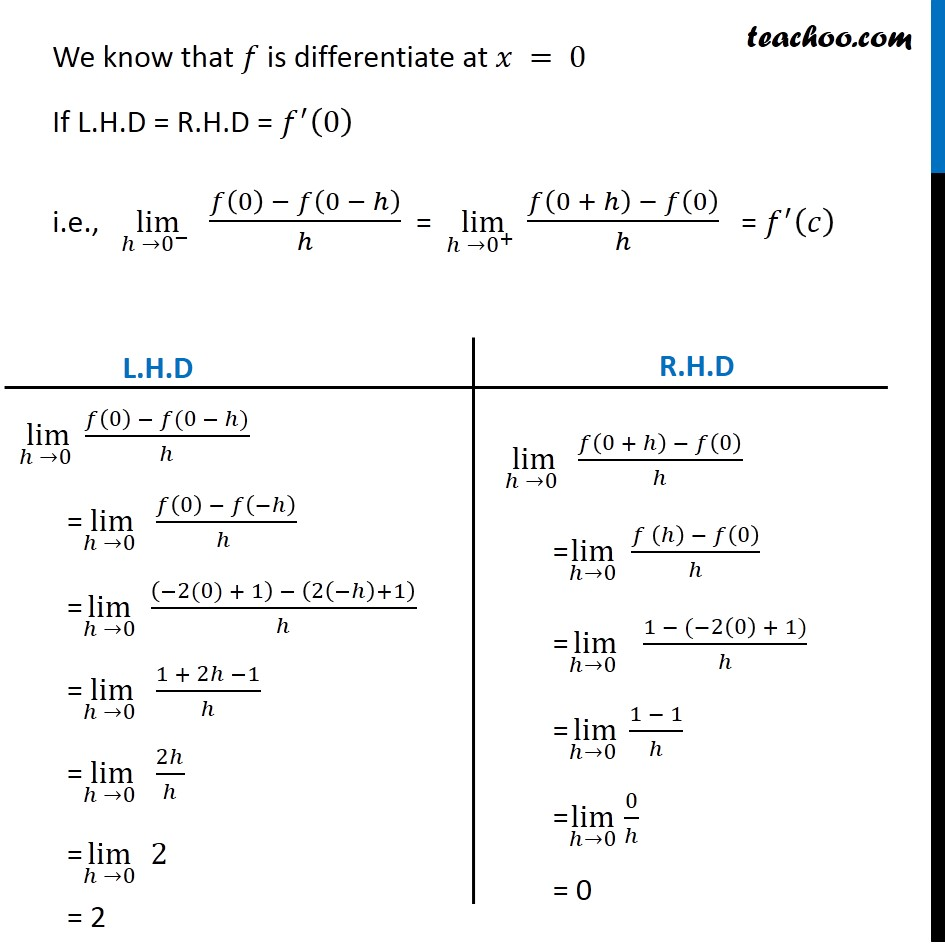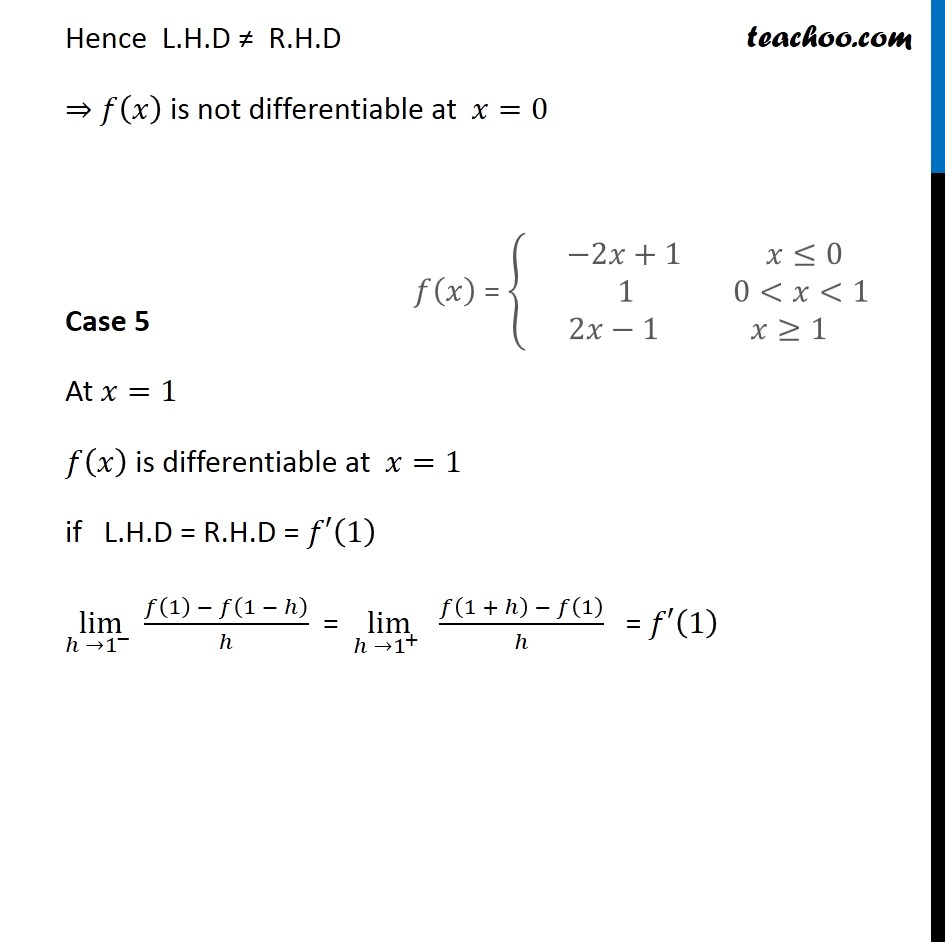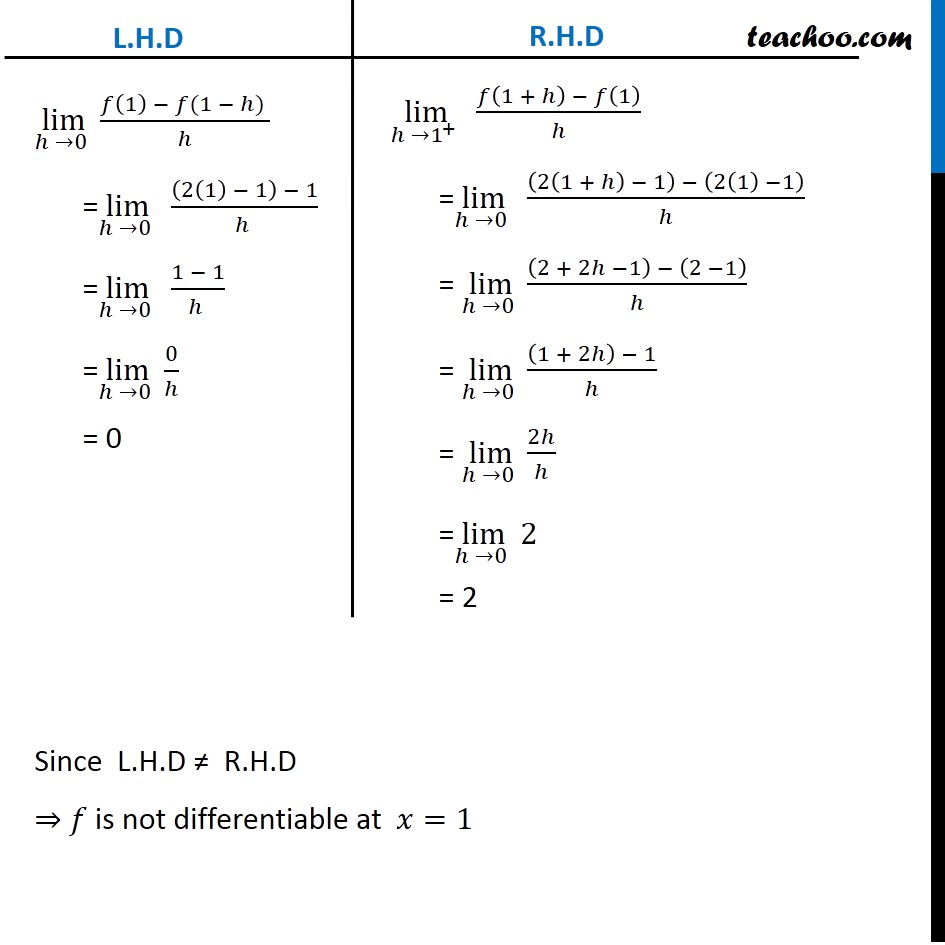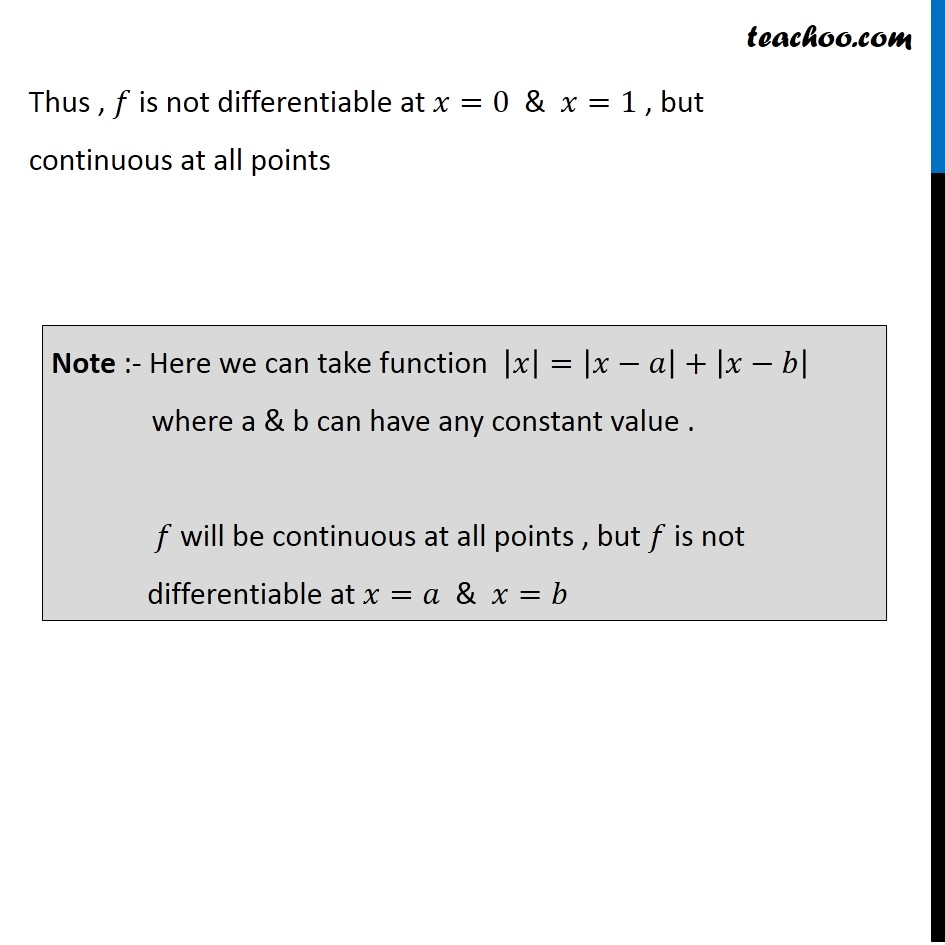1. Chapter 5 Class 12 Continuity and Differentiability
2. Serial order wise
3. Miscellaneous

Transcript

Misc 21 Does there exist a function which is continuous everywhere but not differentiable at exactly two points? Justify your answer. Consider the function 𝑓(𝑥)=|𝑥|+|𝑥−1| 𝑓 is continuous every where , but it is not differentiable at 𝑥 = 0 & 𝑥 = 1 . 𝑓(𝑥)={█( −𝑥−(𝑥−1) 𝑥≤0@𝑥−(𝑥−1) 0<𝑥<1@𝑥+(𝑥−1) 𝑥≥1)┤ = {█( −2𝑥+1 𝑥≤0@ 1 0<𝑥<1@2𝑥−1 𝑥≥1)┤ Checking continuity Case 1 At 𝑥<0 𝑓(𝑥)=−2𝑥+1 𝑓(𝑥) is polynomial ⇒ 𝑓(𝑥) is continuous Case 2 At 𝑥>1 𝑓(𝑥)=2𝑥−1 𝑓(𝑥) is polynomial ⇒ 𝑓(𝑥) is continuous [Every polynomial function is continuous] Case 3 At 0<𝑥<1 𝑓(𝑥)=1 𝑓(𝑥) is constant," " ⇒ 𝑓(𝑥) is continuous Case 4 At 𝑥=0 𝑓(𝑥) = {█( −2𝑥+1 𝑥≤0@ 1 0<𝑥<1@2𝑥−1 𝑥≥1)┤ A function is continuous at 𝑥=0 if LHL = RHL = 𝑓(0) i.e. lim┬(𝑥 →0^− ) 𝑓 (𝑥) = lim┬(𝑥 →0^+ ) 𝑓 (𝑥) = 𝑓(0) & 𝑓(𝑥)= −2𝑥+1 𝑓(0)= −2(0)+1= 1 Hence LHL = RHL = f (0) ⇒ 𝑓 is continuous . lim┬(𝑥 →0^− ) 𝑓 (𝑥) =lim┬(ℎ →0^− ) 𝑓 (−2𝑥+1) Putting 𝑥 = 0 = −2(0)+1 = 1 lim┬(𝑥 →0^+ ) 𝑓 (𝑥) =lim┬(𝑥 →0^+ ) 1 = 1 Case 5 At 𝑥=1 A function is continuous at 𝑥=1 if LHL = RHL = 𝑓(1) i.e. lim┬(𝑥 →1^− ) 𝑓 (𝑥) = lim┬(𝑥 →1^+ ) 𝑓 (𝑥) = 𝑓(1) lim┬(𝑥 →1^− ) 𝑓 (𝑥) =lim┬(𝑥 →1^− )1 = 1 (𝐴𝑠 𝑥<1) lim┬(𝑥 →1^+ ) 𝑓 (𝑥) =lim┬(𝑥 →1^+ ) 2𝑥−1 Putting 𝑥 =1 = 2(1)−1 = 2−1 = 1 (𝐴𝑠 𝑥>1) & 𝑓 (𝑥)=2𝑥−1 𝑓 (1)=2(1)−1=2−1 =1 Hence LHL = RHL = 𝑓(1) ⇒ 𝑓 is continuous at 𝑥=1 Thus 𝑓(𝑥)=|𝑥|+|𝑥−1| is continuous for all value of 𝑥 . To check differentiability Case 1 At 𝑥<1 𝑓(𝑥)=−2𝑥+1 𝑓(𝑥) is polynomial ⇒ 𝑓(𝑥) is differentiable Case 2 At 𝑥>1 𝑓(𝑥)=2𝑥−1 𝑓(𝑥) is polynomial ⇒ 𝑓(𝑥) is differentiable Case 3 At 0<𝑥<1 𝑓(𝑥)=1 𝑓(𝑥) is constant ⇒ 𝑓(𝑥) is differentiable Case 4 At 𝑥=0 𝑓(𝑥) = {█( −2𝑥+1 𝑥≤0@ 1 0<𝑥<1@2𝑥−1 𝑥≥1)┤ We know that 𝑓 is differentiate at 𝑥 = 0 If L.H.D = R.H.D = 𝑓^′ (0) i.e., lim┬(ℎ →0^− ) (𝑓(0) − 𝑓(0 − ℎ))/ℎ = lim┬(ℎ →0^+ ) (𝑓(0 + ℎ) − 𝑓(0))/ℎ = 𝑓^′ (𝑐) lim┬(ℎ →0 ) (𝑓(0) − 𝑓(0 − ℎ))/ℎ =lim┬(ℎ →0 ) (𝑓(0) − 𝑓(−ℎ))/(ℎ ) =lim┬(ℎ →0) ((−2(0) + 1) − (2(−ℎ)+1))/ℎ =lim┬(ℎ →0 ) (1 + 2ℎ −1)/ℎ =lim┬(ℎ →0 ) 2ℎ/ℎ =lim┬(ℎ →0) 2 = 2 lim┬(ℎ →0 ) (𝑓(0 + ℎ) − 𝑓(0))/ℎ =lim┬(ℎ→0) (𝑓 (ℎ) − 𝑓(0))/ℎ =lim┬(ℎ→0 ) (1 − (−2(0) + 1))/ℎ =lim┬(ℎ→0 ) (1 − 1)/ℎ =lim┬(ℎ→0) 0/ℎ = 0 Hence L.H.D ≠ R.H.D ⇒ 𝑓(𝑥) is not differentiable at 𝑥=0 Case 5 At 𝑥=1 𝑓(𝑥) is differentiable at 𝑥=1 if L.H.D = R.H.D = 𝑓′(1) lim┬(ℎ →1^− ) (𝑓(1) − 𝑓(1 − ℎ))/ℎ = lim┬(ℎ →1^+ ) (𝑓(1 + ℎ) − 𝑓(1))/ℎ = 𝑓′(1) 𝑓(𝑥) = {█( −2𝑥+1 𝑥≤0@ 1 0<𝑥<1@2𝑥−1 𝑥≥1)┤ lim┬(ℎ →0 ) (𝑓(1) − 𝑓(1 − ℎ) )/ℎ =lim┬(ℎ →0 ) ((2(1) − 1) − 1)/(ℎ ) =lim┬(ℎ →0 ) (1 − 1)/(ℎ ) =lim┬(ℎ →0 ) 0/ℎ = 0 lim┬(ℎ →1^+ ) (𝑓(1 + ℎ) − 𝑓(1))/ℎ =lim┬(ℎ →0 ) ((2(1 + ℎ) − 1) − (2(1) −1))/ℎ = lim┬(ℎ →0 ) ((2 + 2ℎ −1) − (2 −1))/ℎ = lim┬(ℎ →0 ) ((1 + 2ℎ) − 1)/ℎ = lim┬(ℎ →0 ) 2ℎ/ℎ =lim┬(ℎ →0 ) 2 = 2 Thus , 𝑓 is not differentiable at 𝑥=0 & 𝑥=1 , but continuous at all points Note :- Here we can take function |𝑥|=|𝑥−𝑎|+|𝑥−𝑏| where a & b can have any constant value . 𝑓 will be continuous at all points , but 𝑓 is not differentiable at 𝑥=𝑎 & 𝑥=𝑏

Miscellaneous